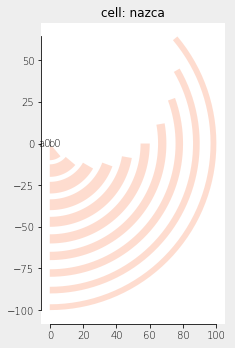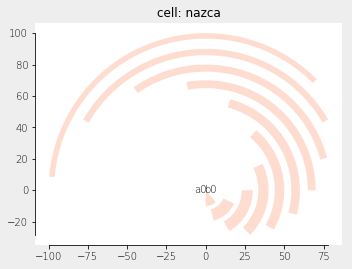Home Forums Nazca Questions and Answers Concentric circles Reply To: Concentric circles

#5674

Dear Gozde,

You can use Nazca Polygon objects for that, which you feed with “normal” polygons [(x1, y1), (x2, y2), … ]. There is a helper module nazca.geometries with methods which generate polygons for various shapes to make life easier.

``````import nazca as nd
import nazca.geometries as geom

for i in range(10):
points = geom.arc(radius=10*i, width=8-0.5*i, angle=40+10*i, N=50)
nd.Polygon(points=points, layer=1).put(0)

nd.export_plt()``````With some extra rotation:

``````for i in range(10):
points = geom.arc(radius=10+10*i, width=8-0.5*i, angle=40+10*i, N=50)
nd.Polygon(points=points, layer=1).put(0, 0, i*15)

nd.export_plt()``````Ronald